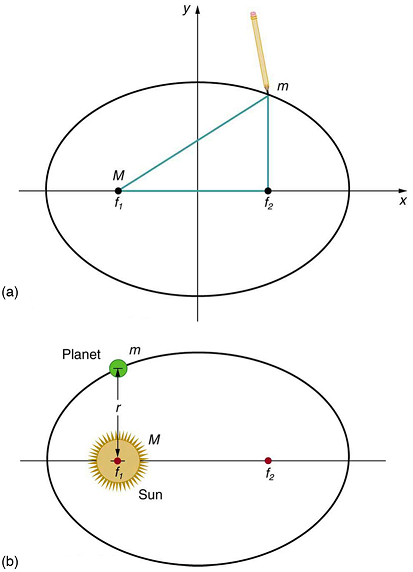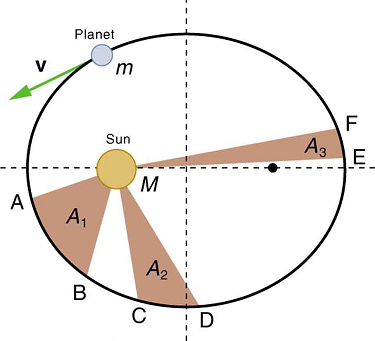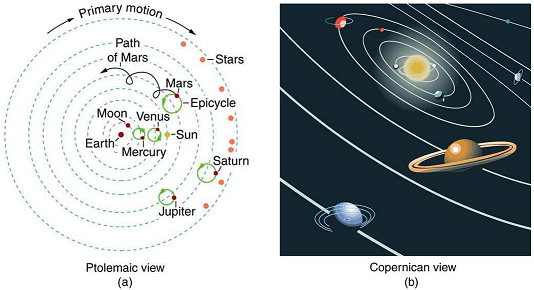$$\require{cancel}$$

6.6: Satellites and Kepler’s Laws: An Argument for Simplicity

•• Contributed by OpenStax
• General Physics at OpenStax CNX

Examples of gravitational orbits abound. Hundreds of artificial satellites orbit Earth together with thousands of pieces of debris. The Moon’s orbit about Earth has intrigued humans from time immemorial. The orbits of planets, asteroids, meteors, and comets about the Sun are no less interesting. If we look further, we see almost unimaginable numbers of stars, galaxies, and other celestial objects orbiting one another and interacting through gravity.

All these motions are governed by gravitational force, and it is possible to describe them to various degrees of precision. Precise descriptions of complex systems must be made with large computers. However, we can describe an important class of orbits without the use of computers, and we shall find it instructive to study them. These orbits have the following characteristics:

1. A small mass $$m$$orbits a much larger mass $$M$$. This allows us to view the motion as if $$M$$ were stationary—in fact, as if from an inertial frame of reference placed on $$M$$ —without significant error. Mass $$m$$ is the satellite of $$M$$, if the orbit is gravitationally bound.
2. The system is isolated from other masses. This allows us to neglect any small effects due to outside masses.

The conditions are satisfied, to good approximation, by Earth’s satellites (including the Moon), by objects orbiting the Sun, and by the satellites of other planets. Historically, planets were studied first, and there is a classical set of three laws, called Kepler’s laws of planetary motion, that describe the orbits of all bodies satisfying the two previous conditions (not just planets in our solar system). These descriptive laws are named for the German astronomer Johannes Kepler (1571–1630), who devised them after careful study (over some 20 years) of a large amount of meticulously recorded observations of planetary motion done by Tycho Brahe (1546–1601). Such careful collection and detailed recording of methods and data are hallmarks of good science. Data constitute the evidence from which new interpretations and meanings can be constructed.

Kepler’s Laws of Planetary Motion

Kepler's First Law

The orbit of each planet about the Sun is an ellipse with the Sun at one focus.Figure $$\PageIndex{1}$$: (a) An ellipse is a closed curve such that the sum of the distances from a point on the curve to the two foci $$(f_1 and f_2)$$ is a constant. You can draw an ellipse as shown by putting a pin at each focus, and then placing a string around a pencil and the pins and tracing a line on paper. A circle is a special case of an ellipse in which the two foci coincide (thus any point on the circle is the same distance from the center). (b) For any closed gravitational orbit, $$m$$ follows an elliptical path with $$M$$ at one focus. Kepler’s first law states this fact for planets orbiting the Sun.

Kepler's Second Law

Each planet moves so that an imaginary line drawn from the Sun to the planet sweeps out equal areas in equal times (see Figure).

Kepler's Third Law

The ratio of the squares of the periods of any two planets about the Sun is equal to the ratio of the cubes of their average distances from the Sun. In equation form, this is $\dfrac{T_1^2}{T_2^2} =\dfrac{r_1^3}{r_2^3}$

where $$T$$ is the period (time for one orbit) and $$r$$ is the average radius. This equation is valid only for comparing two small masses orbiting the same large one. Most importantly, this is a descriptive equation only, giving no information as to the cause of the equality.Figure $$\PageIndex{2}$$: The shaded regions have equal areas. It takes equal times for $$m$$ to go from A to B, from C to D, and from E to F. The mass $$m$$ moves fastest when it is closest to $$M$$. Kepler’s second law was originally devised for planets orbiting the Sun, but it has broader validity.

Note again that while, for historical reasons, Kepler’s laws are stated for planets orbiting the Sun, they are actually valid for all bodies satisfying the two previously stated conditions.

Example $$\PageIndex{1}$$: Find the Time for One Orbit of an Earth Satellite

Given that the Moon orbits Earth each 27.3 d and that it is an average distance of $$3.84 \times 10^8 \, m$$ from the center of Earth, calculate the period of an artificial satellite orbiting at an average altitude of 1500 km above Earth’s surface.

Strategy

The period, or time for one orbit, is related to the radius of the orbit by Kepler’s third law, given in mathematical form in $$\frac{T_1^2}{T_2^2} =\frac{r_1^3}{r_2^3}$$. Let us use the subscript 1 for the Moon and the subscript 2 for the satellite. We are asked to find $$T_2$$. The given information tells us that the orbital radius of the Moon is $$r_1 = 3.84 \times 10^8 \, m$$, and that the period of the Moon is $$T_1 = 27.3 \, d$$.

The height of the artificial satellite above Earth’s surface is given, and so we must add the radius of Earth (6380 km) to get $$r_2 = (1500 +6380) km = 7880 \, km$$. Now all quantities are known, and so $$T_2$$ can be found.

Solution

Kepler’s third law is $\dfrac{T_1^2}{T_2^2} =\dfrac{r_1^3}{r_2^3}.$

To solve for $$T_2$$, we cross-multiply and take the square root, yielding

$T_2^2 = T_1^2 \left(\dfrac{r_2}{r_1} \right)^3$

$T_2 = T_1 \left(\dfrac{r_2}{r_1} \right)^{\frac{3}{2}}.$

Substituting known values yields

$T_2 = 27.3 \, d \times \dfrac {24 \, h}{d} \times \left( \dfrac {7880 \, km}{3.84 \times 10^5 km} \right )^{ \frac{3}{2}}$

$= 1.93 \, h.$

Discussion This is a reasonable period for a satellite in a fairly low orbit. It is interesting that any satellite at this altitude will orbit in the same amount of time. This fact is related to the condition that the satellite’s mass is small compared with that of Earth.

People immediately search for deeper meaning when broadly applicable laws, like Kepler’s, are discovered. It was Newton who took the next giant step when he proposed the law of universal gravitation. While Kepler was able to discover what was happening, Newton discovered that gravitational force was the cause.

Derivation of Kepler’s Third Law for Circular Orbits

We shall derive Kepler’s third law, starting with Newton’s laws of motion and his universal law of gravitation. The point is to demonstrate that the force of gravity is the cause for Kepler’s laws (although we will only derive the third one).

Let us consider a circular orbit of a small mass $$m$$ around a large mass $$M$$, satisfying the two conditions stated at the beginning of this section. Gravity supplies the centripetal force to mass $$m$$. Starting with Newton’s second law applied to circular motion,

$F_{net} = ma_c = m\dfrac{v^2}{r}.$

The net external force on mass $$m$$ is gravity, and so we substitute the force of gravity for $$F_{net}$$:

$G\dfrac{mM}{r^2} = m\dfrac{v^2}{r}.$

The mass $$m$$ cancels, yielding

$G\dfrac{M}{r} = v^2.$

The fact that $$m$$ cancels out is another aspect of the oft-noted fact that at a given location all masses fall with the same acceleration. Here we see that at a given orbital radius $$r$$, all masses orbit at the same speed. (This was implied by the result of the preceding worked example.) Now, to get at Kepler’s third law, we must get the period $$T$$ into the equation. By definition, period $$T$$ is the time for one complete orbit. Now the average speed $$v$$ is the circumference divided by the period—that is,

$v = \dfrac{2\pi r}{T}.$

Substituting this into the previous equation gives $G\dfrac{M}{r} = \dfrac{4\pi^2 r^2}{T^2}.$

Solving for $$T^2$$ yields $T^2 = \dfrac{4\pi^2}{GM}r^3.$

Using subscripts 1 and 2 to denote two different satellites, and taking the ratio of the last equation for satellite 1 to satellite 2 yields

$\dfrac{T_1^2}{T_2^2} = \dfrac{r_1^3}{r_2^3}.$

This is Kepler’s third law. Note that Kepler’s third law is valid only for comparing satellites of the same parent body, because only then does the mass of the parent body $$M$$ cancel.

Now consider what we get if we solve $$T^2 = \frac{4\pi^2}{GM}r^3$$ for the ratio $$r^3/T^2$$. We obtain a relationship that can be used to determine the mass $$M$$ of a parent body from the orbits of its satellites:

$\dfrac{r^3}{T^2} = \dfrac{G}{4\pi^2}M.$

If $$r$$ and $$T$$ are known for a satellite, then the mass $$M$$ of the parent can be calculated. This principle has been used extensively to find the masses of heavenly bodies that have satellites. Furthermore, the ratio $$r^3/T^2$$ should be a constant for all satellites of the same parent body (because $$r^3/T^2 = GM/4\pi$$. (See Table).

It is clear from Table that the ratio of $$r^3/T^2$$ is constant, at least to the third digit, for all listed satellites of the Sun, and for those of Jupiter. Small variations in that ratio have two causes—uncertainties in the $$r$$ and $$T$$ data, and perturbations of the orbits due to other bodies. Interestingly, those perturbations can be—and have been—used to predict the location of new planets and moons. This is another verification of Newton’s universal law of gravitation.

MAKING CONNECTIONS

Newton’s universal law of gravitation is modified by Einstein’s general theory of relativity, as we shall see in Particle Physics. Newton’s gravity is not seriously in error—it was and still is an extremely good approximation for most situations. Einstein’s modification is most noticeable in extremely large gravitational fields, such as near black holes. However, general relativity also explains such phenomena as small but long-known deviations of the orbit of the planet Mercury from classical predictions.

The Case for Simplicity

The development of the universal law of gravitation by Newton played a pivotal role in the history of ideas. While it is beyond the scope of this text to cover that history in any detail, we note some important points. The definition of planet set in 2006 by the International Astronomical Union (IAU) states that in the solar system, a planet is a celestial body that:

1. is in orbit around the Sun,
2. has sufficient mass to assume hydrostatic equilibrium and
3. has cleared the neighborhood around its orbit.

A non-satellite body fulfilling only the first two of the above criteria is classified as “dwarf planet.”

In 2006, Pluto was demoted to a ‘dwarf planet’ after scientists revised their definition of what constitutes a “true” planet.

ParentSatelliteAverage orbital radius r(km)Period T(y)$$r^3/T^2 (km^3/y^2)$$
EarthMoon

$$3.84 \times 10^5$$

3.84×105
0.07481

$$1.01 \times 10^{19}$$

1.01×1019
SunMercury

$$5.79 \times 10^7$$ 5.79

0.2409

$$3,34 \times 10^{24}$$

3.34×1024
Venus

$$1.082 \times 10^8$$

1.082×108
0.6150

$$3.35 \times 10^{24}$$

3.35×1024
Earth

$$1.496 \times 10^8$$

1.496×108
1.000

$$3.35 \times 10^{24}$$

3.35×1024
Mars

$$2.279 \times 10^8$$

2.279×108
1.881

$$3.35 \times 10^{24}$$

3.35×1024
Jupiter

$$7.783 \times 10^8$$

7.783×108
11.86

$$3.35 \times 10^{24}$$

3.35×1024
Saturn

$$1.427 \times 10^9$$

1.427×109
29.46

$$3.35 \times 10^{24}$$

3.35×1024
Neptune

$$4.497 \times 10^9$$

4.497×109
164.8

$$3.35 \times 10^{24}$$

3.35×1024
Pluto

$$5.90 \times 10^9$$

5.90×109
248.3

$$3.33 \times 10^{24}$$

3.33×1024
JupiterIo

$$4.22 \times 10^5$$

4.22×105
0.00485 (1.77 d)

$$3.19 \times 10^{21}$$

3.19×1021
Europa

$$6.71 \times 10^5$$

6.71×105
0.00972 (3.55 d)

$$3.20 \times 10^{21}$$

3.20×1021
Ganymede

$$1.07 \times 10^6$$

1.07×106
0.0196 (7.16 d)

$$3.19 \times 10^{21}$$

3.19×1021
Callisto

$$1.88 \times 10^6$$

1.88×106
0.0457 (16.19 d)

$$3.20 \times 10^{21}$$

3.20×1021

The universal law of gravitation is a good example of a physical principle that is very broadly applicable. That single equation for the gravitational force describes all situations in which gravity acts. It gives a cause for a vast number of effects, such as the orbits of the planets and moons in the solar system. It epitomizes the underlying unity and simplicity of physics.

Before the discoveries of Kepler, Copernicus, Galileo, Newton, and others, the solar system was thought to revolve around Earth as shown in Figure (a). This is called the Ptolemaic view, for the Greek philosopher who lived in the second century AD. This model is characterized by a list of facts for the motions of planets with no cause and effect explanation. There tended to be a different rule for each heavenly body and a general lack of simplicity.

Figure (b) represents the modern or Copernican model. In this model, a small set of rules and a single underlying force explain not only all motions in the solar system, but all other situations involving gravity. The breadth and simplicity of the laws of physics are compelling. As our knowledge of nature has grown, the basic simplicity of its laws has become ever more evident.Figure $$\PageIndex{3}$$: (a) The Ptolemaic model of the universe has Earth at the center with the Moon, the planets, the Sun, and the stars revolving about it in complex superpositions of circular paths. This geocentric model, which can be made progressively more accurate by adding more circles, is purely descriptive, containing no hints as to what are the causes of these motions. (b) The Copernican model has the Sun at the center of the solar system. It is fully explained by a small number of laws of physics, including Newton’s universal law of gravitation.

Summary

• Kepler’s laws are stated for a small mass $$m$$ orbiting a larger mass $$M$$ in near-isolation. Kepler’s laws of planetary motion are then as follows:

Kepler’s first law

The orbit of each planet about the Sun is an ellipse with the Sun at one focus.

Kepler’s second law

Each planet moves so that an imaginary line drawn from the Sun to the planet sweeps out equal areas in equal times.

Kepler’s third law

The ratio of the squares of the periods of any two planets about the Sun is equal to the ratio of the cubes of their average distances from the Sun:

$\dfrac{T_1^2}{T_2^2} =\dfrac{r_1^3}{r_2^3},$

where T is the period (time for one orbit) and $$r$$ is the average radius of the orbit.

• The period and radius of a satellite’s orbit about a larger body M are related by

$T^2=\frac{4π^2}{GM}r^3$ or $\dfrac{r^3}{T^2} = \dfrac{G}{4\pi^2}M.$

Contributors

Paul Peter Urone (Professor Emeritus at California State University, Sacramento) and Roger Hinrichs (State University of New York, College at Oswego) with Contributing Authors: Kim Dirks (University of Auckland) and Manjula Sharma (University of Sydney). This work is licensed by OpenStax University Physics under a Creative Commons Attribution License (by 4.0).